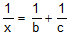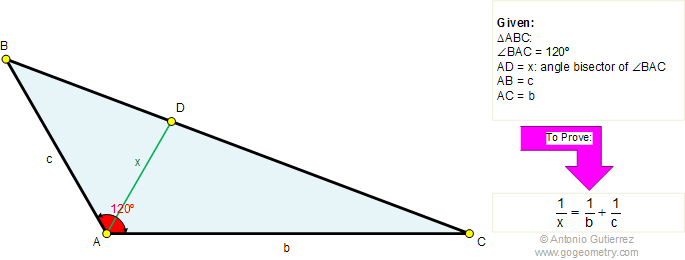# Online Geometry Problem 304. Triangle, Angle bisector, 120 Degrees. Level: High School, College, Mathematics Education

 In a triangle ABC, the angle BAC is equal to 120 degrees. If AB = c, AC = b, and AD = x. Prove that. (x is one-half the harmonic mean of b and c)Home | Search | Geometry | Problems | All Problems | Open Problems | Visual Index | 301-310 | Parallelogram Midpoint | Similarity, Ratios, Proportions | Harmonic Mean View or post a solution